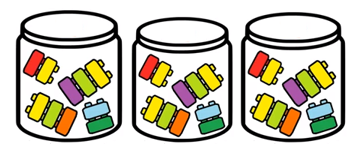Mathematics
Easy

Question

# Each jar has 10 blocks. Find the total blocks.## 30407920Hint:

## The correct answer is: 30

### Here, there are some jars and each jar contain 10 blocks. We have to find the total number of blocks.Number of blocks in each jar =10. Total number of jars = 3.So, total number of blocks = 10×3 = 30.hence, the correct option is

Multiplication is repetitive addition. So, we could just add 10 3 times.

### Related Questions to study#### With Turito Foundation.#### Get an Expert Advice From Turito.